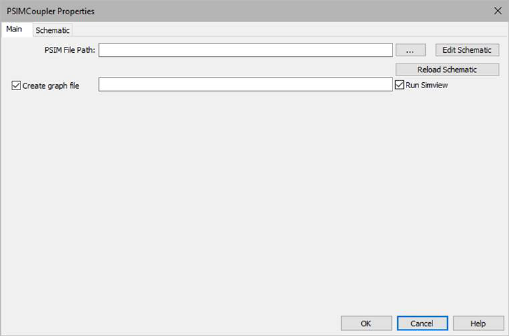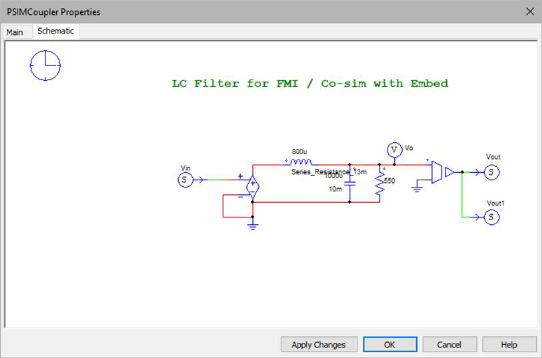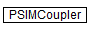## Current Controller

Category: Toolbox > eDrives > Controllers

Inputs:

freq_n: Normalized frequency reference. Scaled Integer (2.32)

id_ref: Normalized d-axis current reference. Scaled Integer (2.32)

i_a: Normalized measured alpha-axis current. Scaled Integer (2.32)

i_b: Normalized measured beta-axis current. Scaled Integer (2.32)

udc_n: Normalized measured DC bus voltage. Scaled Integer (2.32)

cntr_on: Controller enable. (binary input).

Outputs:

mA_ref: Modulation index reference for phase A. Scaled Integer (8.32)

mB_ref: Modulation index reference for phase B. Scaled Integer (8.32)

mC_ref: Modulation index reference for phase C. Scaled Integer (8.32)

Description: The Current Controller block uses the error between reference current signals and measured current signals (in d,q coordinate frame) to generate modulation indices for three-phase motor converters.

All inputs are divided by their respective full-scale values to derive normalized values. For example, id_n = id_ref/C_fs, where id_ref is the user input and C_fs  is the full-scale current. The outputs are modulation indices. For example,, whereis the reference average voltage/per sample for phase A andis the measured DC bus voltage of the converter.Current Controller Kp (V/A): Indicates the proportional gain for the current loop.

Current Controller wi (rad/s): Indicates the bandwidth of the current controller.

Fullscale Current (A): Indicates the full-scale current value for normalization.

Fullscale Freq (Hz): Indicates the full-scale frequency value for normalization.

Fullscale Voltage (V): Indicates the full-scale voltage value for normalization.

Max Mod Index: Indicates the maximum modulation index value (absolute).

User Current Ramp: Indicates the safety limits to avoid sudden jerks in the input values.

User Frequency Ramp: Indicates the safety limit to avoid sudden jerks in the input frequency value.